﻿ A Common Fixed Point Theorem for Pair of Mappings in Cone Metric Spaces

### A Common Fixed Point Theorem for Pair of Mappings in Cone Metric Spaces

K. Prudhvi

Journal of Mathematical Sciences and Applications

## A Common Fixed Point Theorem for Pair of Mappings in Cone Metric Spaces

Department of Mathematics, University College of Science, Saifabad, Osmania University, Hyderabad, Telangana State, India

### Abstract

In this paper, we prove a fixed point theorem for pair of mappings in cone metric spaces, with using the normality. Our result is generalization of some recent results existing in the literature.

• K. Prudhvi. A Common Fixed Point Theorem for Pair of Mappings in Cone Metric Spaces. Journal of Mathematical Sciences and Applications. Vol. 5, No. 1, 2017, pp 17-18. http://pubs.sciepub.com/jmsa/5/1/2
• Prudhvi, K.. "A Common Fixed Point Theorem for Pair of Mappings in Cone Metric Spaces." Journal of Mathematical Sciences and Applications 5.1 (2017): 17-18.
• Prudhvi, K. (2017). A Common Fixed Point Theorem for Pair of Mappings in Cone Metric Spaces. Journal of Mathematical Sciences and Applications, 5(1), 17-18.
• Prudhvi, K.. "A Common Fixed Point Theorem for Pair of Mappings in Cone Metric Spaces." Journal of Mathematical Sciences and Applications 5, no. 1 (2017): 17-18.

 Import into BibTeX Import into EndNote Import into RefMan Import into RefWorks

### 1. Introduction

In 2007, cone metric space was introduced by Huang and Zhang , they replaced set of real numbers by an ordered Banach space and proved some fixed point theorems for contractive type conditions in cone metric spaces. Subsequently, many authors for e.g. (see, [1, 2, 3, 5]) extended, improved and generalized the results of Huang and Zhang . Recently, Rezapour et. al.  generalized the results of Huang and Zhang  and omitting the normality. In this paper, we proved a fixed point theorem for pair of mappings in cone metric spaces with using the normality. Our result is generalization of the results of Abbas and Jungck .

### 2. Preliminaries

The following are the basic concepts on cone metric space due to Huang and Zhang , which are needful in our main results.

2.1  Definition

Let B be a real Banach space and P be a subset of B, P is called a cone if

(i) P is closed, non-empty and P ≠ {0};

(ii) a, b are non-negative real numbers and x,y∈P ⇒ ax+by∈P;

(iii) P ∩ (-P) = {0}.

For a given cone P ⊆ B, we define a partial ordering ≤ with respect to P by x ≤ y if and only if y-x∈P, x<y stand for x ≤ y and x ≠ y, while x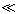y will stand for y-x∈int P, where int P denotes the interior of P.

2.2  Definition

The cone P is called normal if there is a number M >0 such that for all x, y∈M,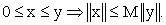The least positive number satisfying the above is called the normal constant of P.

2.3  Definition

Let X be a nonempty set of B. Suppose that the mapping d: X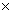X→B, satisfies:

(1) 0 ≤ d(x, y) for all x, y∈X and d(x, y) = 0 if and only if x = y;

(2) d(x, y) = d(y, x) for all x, y∈X;

(3) d(x, y)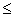d(x, z) + d(z, y) for all x, y, z∈X.

Then d is called a cone metric on X, and (X, d) is called a cone metric space.

It is clear that the concept of a cone metric space is more general than that of a metric space.

2.4  Example

Let B =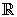2, P = {(x, y)∈B: x, y ≥ 0}⊂2, X =and d: X × X→B such that d(x, y) = (│x - y│, α│x - y│), where α ≥ 0 is a constant. Then (X, d) is a cone metric space.

2.5  Definition

Let (X, d) be a cone metric space. We say that {xn} is

(i) a convergent sequence if for any c>>0, there is a natural number N such that for all n>N, d(xn, x) <<c, for some fixed x in X .We write this xn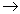x (as n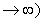.

(ii) a Cauchy sequence if for every c in B with c>>0, there is a natural number N such that for all n, m>N, d(xn,xm)<<c;

The space (X, d) is called a complete cone metric space, if every Cauchy sequence is convergent .

2.6 Definition

Suppose S and T are self-maps of X, if x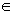X such that S(x) = T(x) = x , then x is called a common fixed point of S and T.

2.7  Definition

Let f and g be self maps of a set X. If w = fx = gx for some x in X, then x is called a coincidence point of f and g, and w is called a point of coincidence of f and g.

2.8  Definition

A pair (f, T) of self-mappings on X is said to be weakly compatible if they commute at their coincidence point (i.e., fTx = Tfx whenever fx = Tx).

2.9  Proposition

Let f and g be weakly compatible self maps of a set X. If f and g have a unique point of coincidence w = fx = gx, then w is a unique common fixed point of f and g.

### 3. Fixed Point Theorem

3.1 Theorem Let (X, d) be a cone metric space, and the mappings f, g: X→X satisfy the contractive condition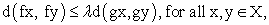(1)

where, λ∈[0, 1) is a constant. If the range of g contains the range of f and g(X) is a complete subspace of X. Moreover, if f and g are weakly compatible, then f and g have a unique common fixed point.

Proof: Let x0 be an arbitrary point in X. Since f(X) ⊆ g(X), then there exists x1 ∈X such that f(x0) = g(x1). By the induction, a sequence {xn} can be chosen such that fxn = gxn+1 (n = 0, 1, 2, 3…). Thus by (1),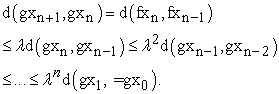Then, for n > m,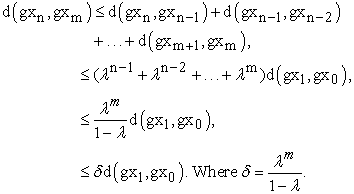(2)

Let 0<< c be given, choose a natural number N1 such that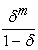d(gx1, gx0) << c , for all m ≥ N1.

Thus, d(gxn , gxm) << c, for n>m.

Therefore, {gxn} is a Cauchy sequence. Since, g(X) is complete, there exist a point q in g(X) such that gxn → q as n → ∞ and there exist p∈X such that gp = q. Hence, by (1), we have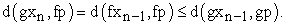Letting, n → ∞ , we get thatTherefore, d(q , fp) = 0.

That is, q = fp.

Therefore, fp = q = gp.

Now, we show that f and g have a unique point of coincidence. For this, assume that there exist another point u in X such that fu = gu.

Thus, by (1), we have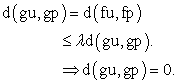That is, gu = gp.

Since, f and g are weakly compatible, f and g have a unique point of coincidence, then by the Proposition (2.9), we get that f and g have a unique common fixed point.

3.4 Remark. In the above Theorem 3.1 with P is a normal cone, then we get that Theorem 2.1 of Abbas and Jungck .

3.5 Remark. If we take g = Ix is the identity map on X and X is a complete metric space in the above Theorem 3.1, then we get that Theorem 2.3 of Rezapour et. al. .

3.6 Remark If we take g = Ix is the identity mapping on X and X is a complete metric space with P is a normal cone in the above Theorem 3.1, then we get that Theorem1 Huang and Zhang .

### 4. Conclusion

Our Theorem 3.1 in the main result is a generalization result of Abbas and Jungck .

### References

  M. Abbas and G. Jungck, Common fixed point results for non commuting mappings without continuity in cone metric spaces, J. Math. Anal. Appl. 341(2008) 416-420.In article  M. Arshad, A. Azam, P. Vetro, Some common fixed point results on cone metrics paces, Fixed Point Theory Appl. (2009)11, Article ID 493965.In article  Guangxing Song, Xiaoyan Sun, Yian Zhao, Guotao Wang, New common fixed point theorems for maps on cone metric spaces, Appl. Math. Lett. 32(2010) 1033-1037.In article View Article  L. G. Huang, X. Zhang, Cone metric spaces and fixed point theorems of contractive mappings, J. Math. Anal. Appl. 332(2) (2007) 1468-1476.In article  D. IIic, V. Rakocevic, Common fixed points for maps on cone metric space, J. Math. Anal. Appl. 341(2008) 876-886.In article  Sh. Rezapour and R. Hamlbarani, Some notes on the paper cone metric spaces and fixed point theorem of contractive mappings, J. Math. Anal. Appl. 345, 200.In article